# 4 Time-to-event endpoints

Time-to-event endpoints may be the most commonly used endpoints applying group sequential designs. There is more flexibility allowed in these designs, which means the interface is a little more complex.

Organization

• Input
• Hazard ratios
• Failure rates
• Enrollment
• Output
• Graphical output
• Tabular summary
• Textual summary
• Making a report
• The ‘Report tab’
• Editing the report
• Integer event counts
• Integer sample size
• Exercises

## 4.1 Overview and input

Time-to-event designs in the gsDesign web application and the underlying gsDesign R package currently focus solely on the proportional hazards model. Key factors determining sample size for these designs are the treatment effect under the alternate hypothesis, how fast events are expected to accumulate (failure rates), and enrollment rates. As you can see in the next figure, when you select “Time-to-event” as your endpoint type, each of these has its own tab in the input window. Thus, time-to-event endpoints have the most complex and flexible design capabilities in gsDesign.

The first figure demonstrates the default input layout.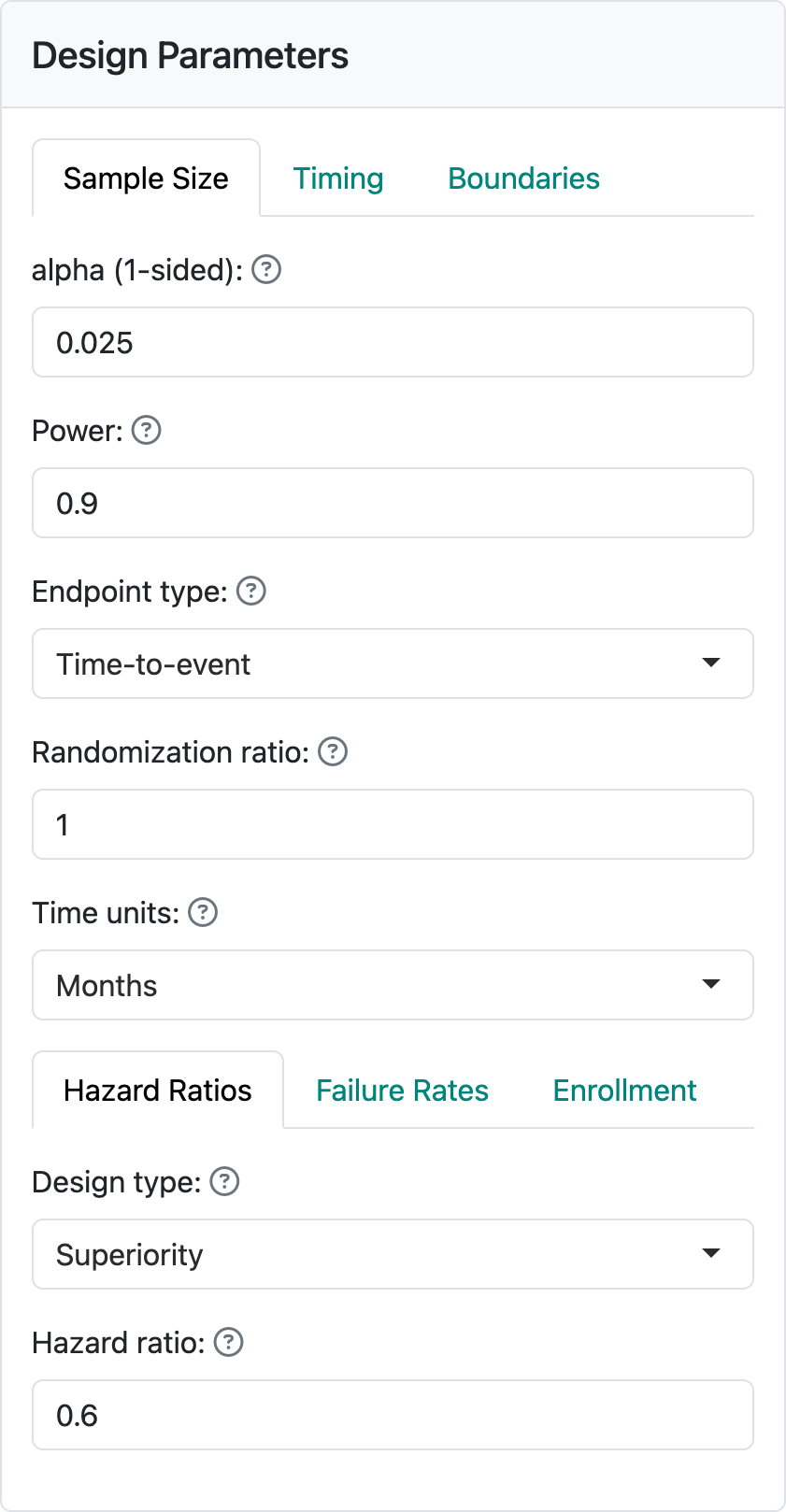### 4.1.1 Hazard ratios

The hazard ratio represents the assumed hazard rate in the experimental group divided by the hazard rate in the control group. Thus, a value less than one indicates a lower hazard for an event in the experimental group, corresponding to a treatment benefit. As previously noted, a constant hazard ratio over time may often not be true, but it is a common assumption both for sample size computation and for analysis using either the logrank test statistic or the Cox proportional hazards model; we will not describe these here, but they are easily found on the internet or in standard texts. You can see a text box to set the assumed hazard ratio at the bottom of the input screen on the lefthand-side of this page. Here, we have assumed a hazard ratio of 0.6.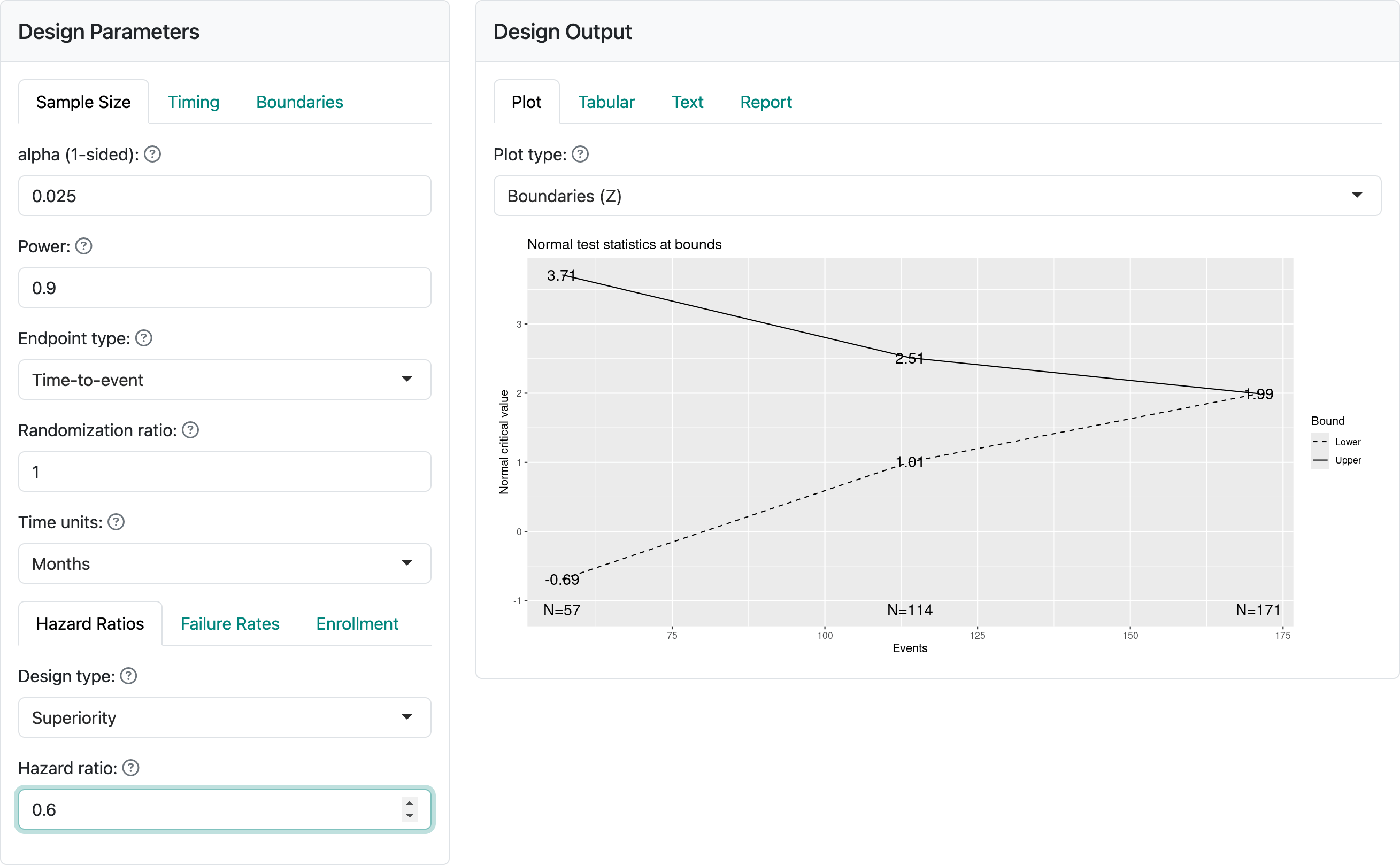By selecting ‘Non-inferiority’, you are allowed to set a hazard ratio both for the null and the alternate hypotheses. Most often, the null hypothesis (H0) hazard ratio is set to 1 which means that you are trying to demonstrate superiority of the experimental treatment compared to control. Non-inferiority, or ruling out an H0 value greater than 1 may be acceptable if you only need to demonstrate that the experimental treatment is ‘close’ too as good as control (or better). The alternate hypothesis H1 hazard ratio should be set to a value less than the null hypothesis. As an example, for new diabetes drugs, it is required to rule out a hazard ratio of 1.3 for cardiovascular endpoints. The above figure indicates an assumption of no difference under the alternate hypothesis H1 and a trial powered to rule out a hazard ratio of 1.3 under the null hypothesis H0.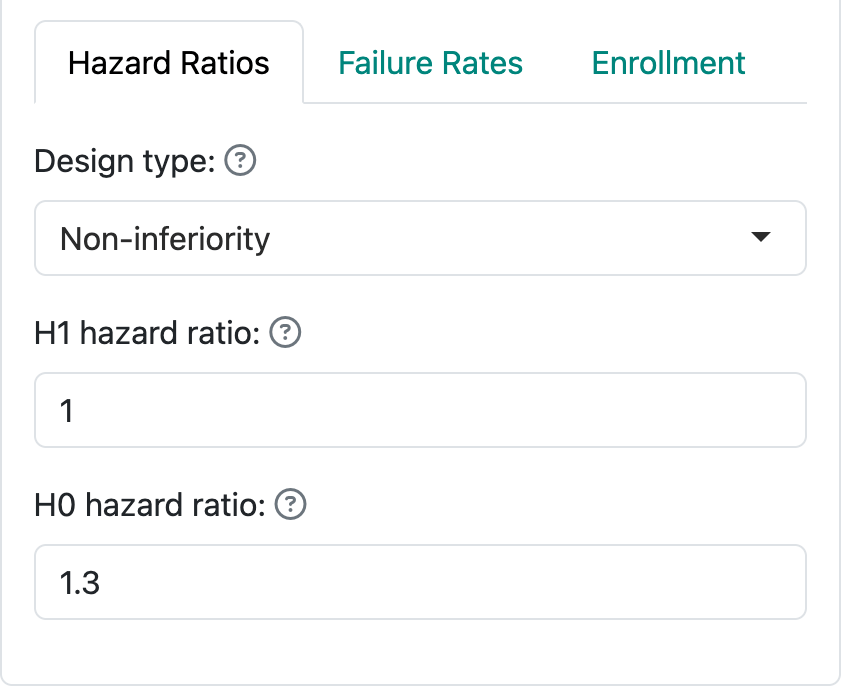### 4.1.2 Failure rates

Failure rates specify the level of risk/rate of events over time. The web interface allows only piecewise constant failure rates, which corresponds to a piecewise exponential distribution for the time until an event. The display below shows the ‘Failure Rates’ tab where you can specify the failure rate for the control group. Note at the top of the figure you can specify a time unit for the distribution with ‘Months’, ‘Weeks’, and ‘Years’ as built in options; this only impacts text in the output.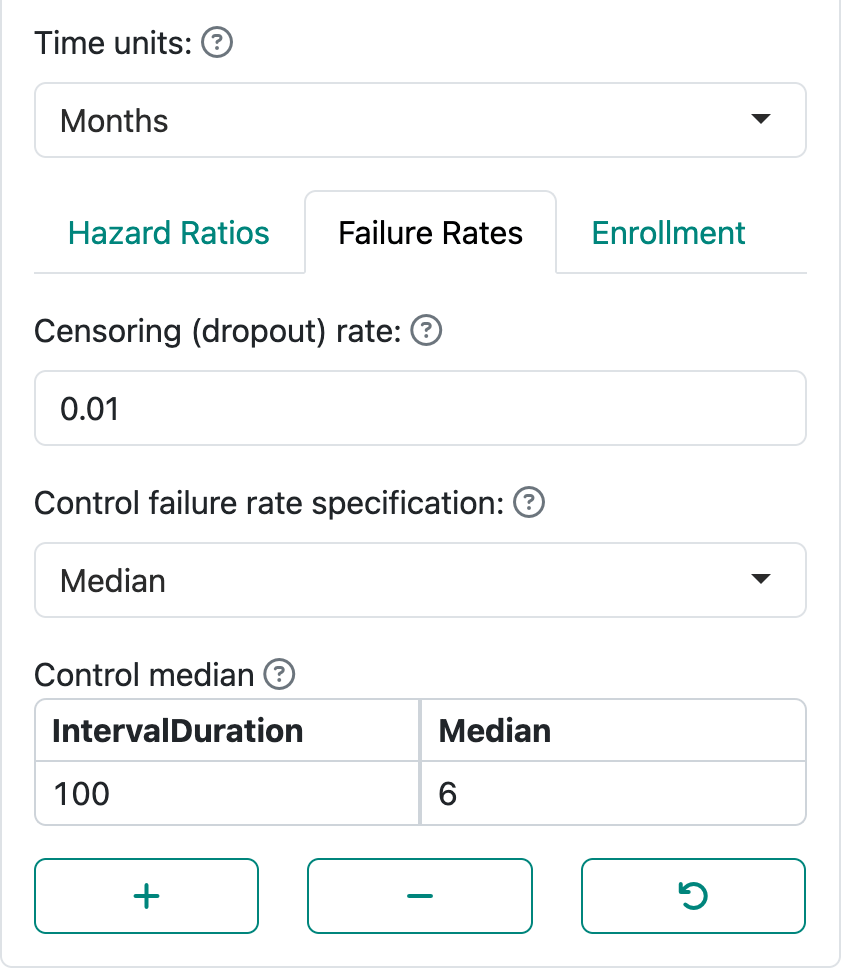‘Control failure rate specification’ allows specification of the failure rate using a ‘Median’ or a ‘Failure rate’. Letting $$\lambda$$ denote the failure rate, the probability that the time to event is greater than a value $$t > 0$$ is $$P(t) = \exp(-\lambda t)$$. A median ($$P(m) = 0.5$$) is simply derived as $$\log(2)/m$$. The above display allows specification of $$m$$. The display below allows specification of the failure rate $$\lambda$$: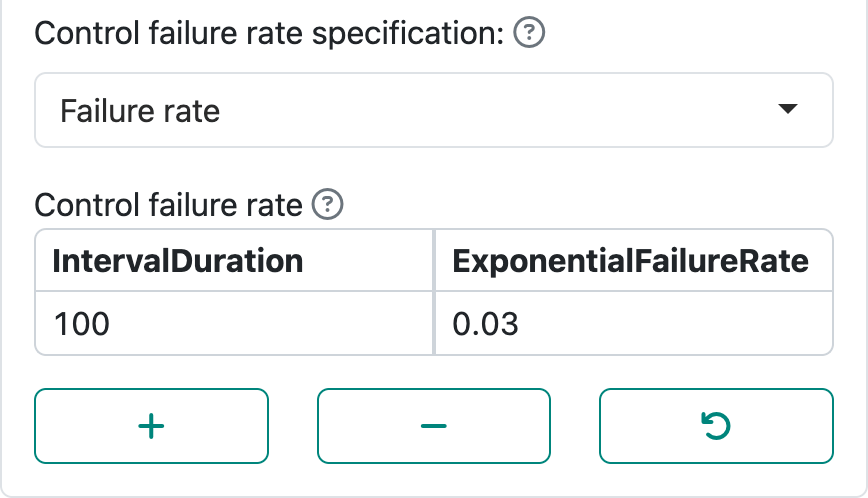By using the ‘+’ and ‘-’ controls you can set up intervals with piecewise constant failure rates. For instance, in an oncology trial control treatment may be expected to have a low failure rate initially followed by expectation of a higher rate after disease has had a chance to progress and finally lowering again after high-risk patients have largely failed. Note that the final row in the table is extended to $$\infty$$. If you had a study where endpoints only count, say, in the first 6 months, the failure rate after month 6 could be set to 0.

The combination of the control failure rate plus the hazard ratio specifies the failure rate for the experimental group under the alternate hypothesis. With the above $$\lambda$$ for the control group and a hazard ratio of $$0.6$$, the experimental group hazard rate under the alternate hypothesis would be $$0.03 \times 0.6 = 0.18$$. Starting with a control median $$m = 6$$ months and hazard ratio of $$0.6$$, the experimental group median is $$6 / 0.6 = 10$$ months.

The ‘Failure Rates’ tab also allows specification of censoring or dropout rates as shown below. This is always done by specifying an exponential dropout rate that is assumed to be common between the control and experimental groups; when using the R package without the web interface, different dropout rates in each group are allowed. Here we have assumed a rate of 0.01 or 1% per month.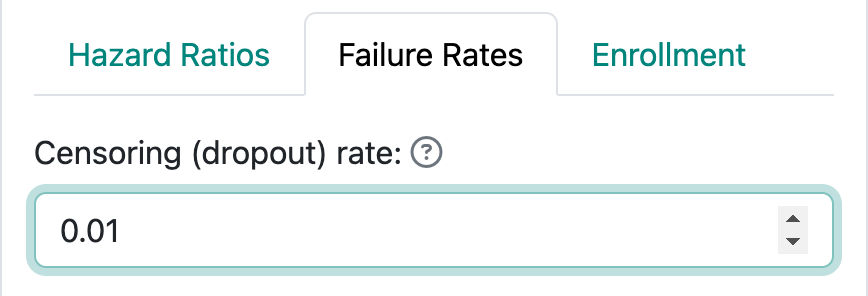### 4.1.3 Enrollment

There are three ways of specifying enrollment assumptions, two of which are recommended over the third: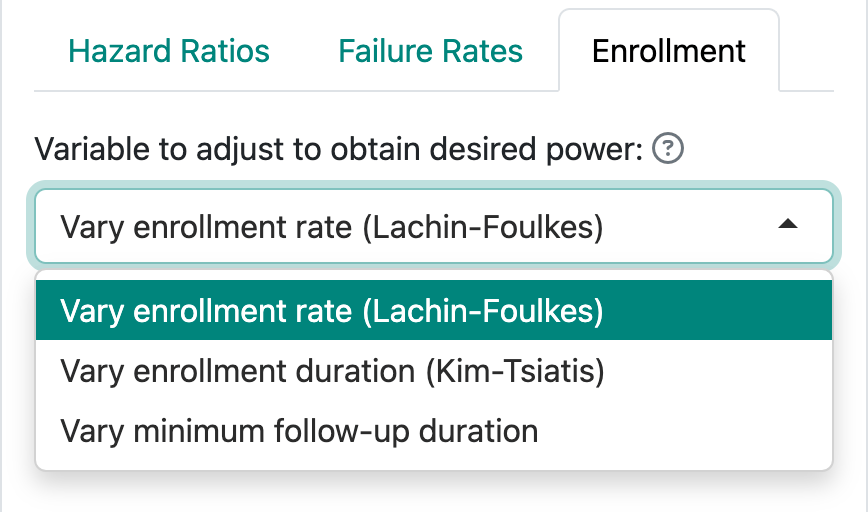1. You can fix the enrollment and follow-up duration and allow the enrollment rate to be computed by gsDesign; this is the John M Lachin and Mary A Foulkes12 method. The underlying calculations for fixed design sample sizes are based on these methods.
2. You can fix the enrollment rate and follow-up duration and allow the enrollment duration to vary. This corresponds to methods suggested by Kyungmann Kim and Anastasios A Tsiatis13.
3. Finally, you can fix the enrollment duration and rate and allow the follow-up duration to vary to obtain the desired power. This method often creates situations that cannot properly power a trial without adjustment of the enrollment duration or rate.

#### 4.1.3.1 Lachin-Foulkes enrollment assumptions

The default enrollment screen is shown in the following figure. This corresponds to the Lachin and Foulkes14 method (option 1 above). Here we have specified 12 months of enrollment with an additional 6 months of follow-up. This results in an assumed total study duration of 12 + 6 = 18 months. We are also allowed to specify an ‘Enrollment ramp-up duration’, which has been set to the first 6 months of enrollment in this case. The enrollment ramp-up period is divided into 3 equally long intervals, 2 months each in this case, during which enrollment rates are assumed to be 25%, 50% and 75% of the final enrollment rate. This is a simplification of what is allowed when coding in R, but was felt to allow sufficient flexibility and a simplification of the user interface.

When the actual sample size is computed using the Lachin and Foulkes method, the specified relative enrollment rates are fixed and the absolute rates are increased or decreased as needed to obtain the specified power.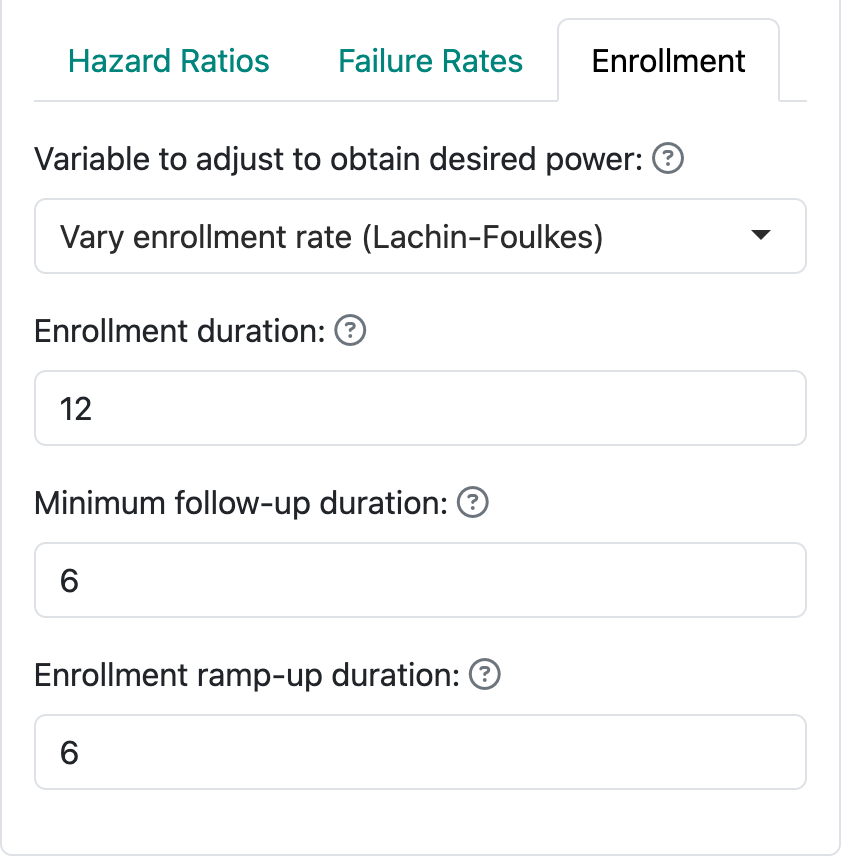#### 4.1.3.2 Kim-Tsiatis enrollment assumptions

In option 2, we follow methods of Kim and Tsiatis15 by fixing the enrollment rates and minimum duration of follow-up, allowing enrollment duration to vary to increase or decrease power to the desired level. The following figure demonstrates a steady state enrollment rate of 10 patients per month (or week or year, depending on the time unit you specify). This continues for a duration computed by the software and is followed by a minimum follow-up specified here as 6 months. The enrollment ramp-up duration works as before.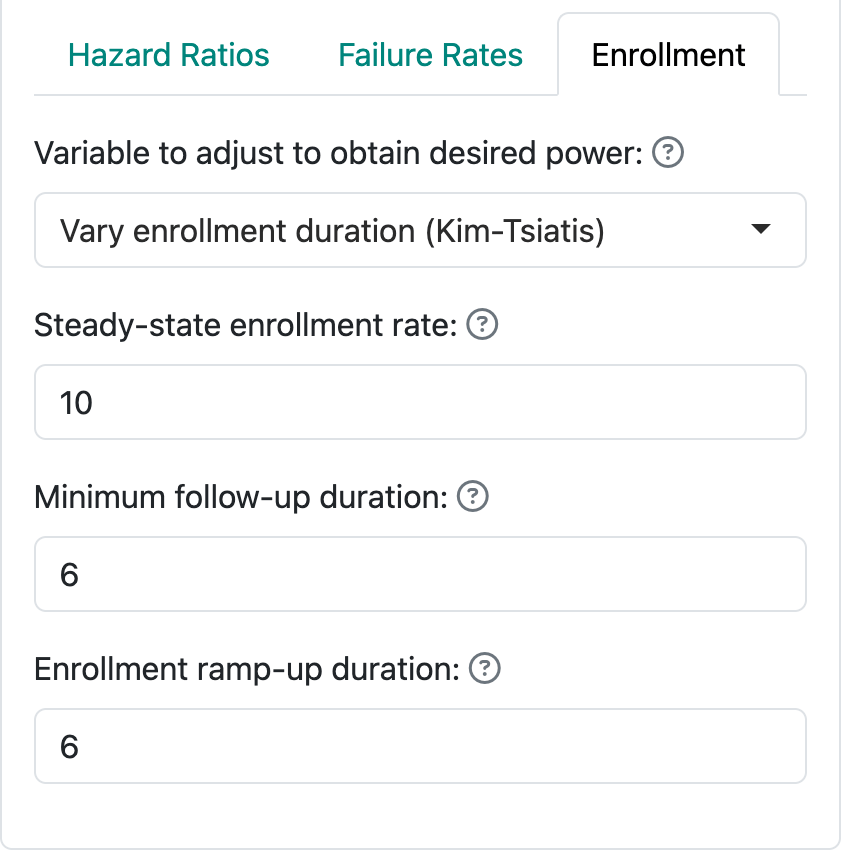#### 4.1.3.3 Vary minimum follow-up duration

The third, less recommended option for specifying enrollment assumptions is shown in the following figure. In this case, we specify the enrollment rate at steady state (10 per month) and enrollment duration (12 months) and allow the follow-up duration to vary in attempt to achieve the desired power. The enrollment ramp-up duration works as before and is specified as 6 months here. Thus, we here assume an enrollment rate of 2.5 patients per month for 2 months, 5 patients per month for 2 months, 7.5 patients for 2 months, and 10 patients per month for the final six months of the enrollment period. This results in a planned enrollment of $$2 \times (2.5 + 5 + 7.5) + 6 \times 10 = 90$$ patients. This helps you realize why this method often fails to produce a solution with the desired power; i.e., more or fewer than 90 patients enrolled over 12 months may be required produce the desired power and Type I error, regardless of the follow-up duration. With defaults of 90% power, 2.5% one-sided Type I error and a hazard ratio of 0.6, for instance, the rates specified here will not work; changing to a longer enrollment duration (e.g., 24 months) or higher steady state enrollment rate (e.g., 25 per month) result in a solution under these assumptions.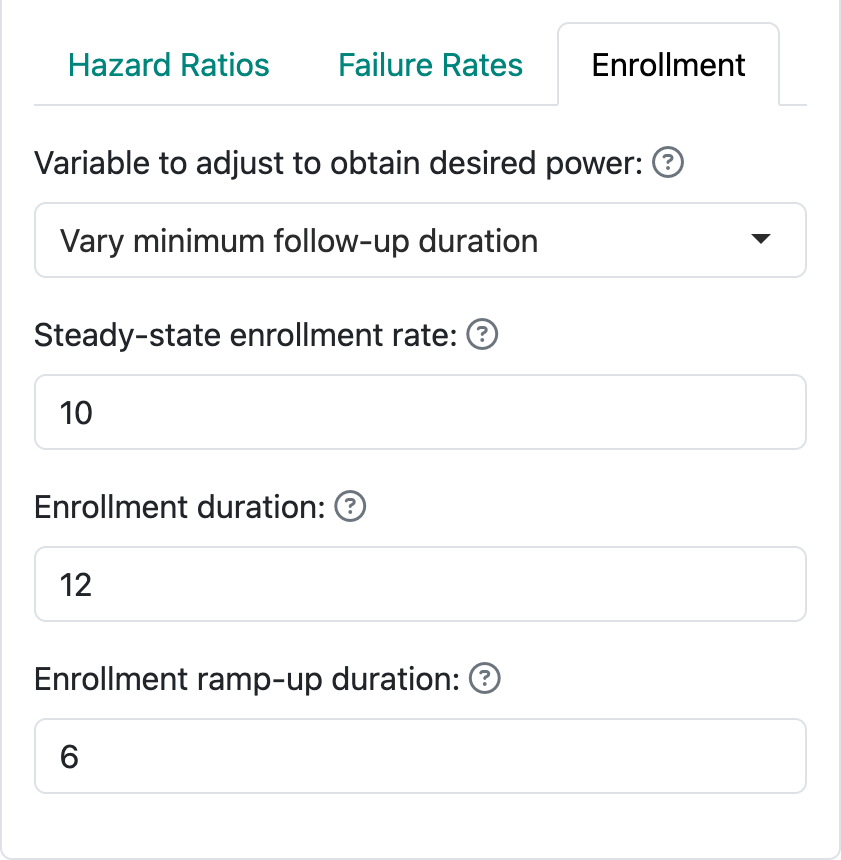## 4.2 Output

### 4.2.1 Tabular output

Tabular output provides a nearly comprehensive summary of a design for a time-to-event study that is easily read and copied into a word processor. Note: the caveat to this copying capability is that you may need to do some table reformatting in your word processor. The example output on the following page was created using the Kim-Tsiatis enrollment option and a control median of 6 months; the latter is not summarized in this output, but probably will be in a future version. The enrollment ramp-up assumption is also not shown here. The enrollment rate assumptions can be found on the ‘Text’ or ‘Code’ tabs and should be recorded if you are copying output to a file saving your design assumptions.

The table created here provides information on each interim analysis and the final analysis. In the left-hand column, the percent of final required events for the interim analyses and the expected enrollment, number of events and calendar time relative to study start when each analysis is performed is shown.

The right-hand columns show properties of the efficacy and futility bounds:

1. $$Z$$-values (normal test statistics) at the bounds
2. Nominal one-sided $$p$$-values at the bounds.
3. The approximate hazard ratio at the bounds.
4. The probability of crossing a bound under the alternate hypothesis ($$H_1$$).
5. The probability of crossing a bound under the null hypothesis.

Using R coding instead of the web interface also allows a summary of conditional or predictive power at the interim bounds.

At the bottom of the table, a text string is printed describing many of the design specifications with the exceptions noted above. Read through this carefully to see what is there. This will change dynamically as you change input options.Reading through this table carefully is key to ensuring you have selected a suitable design:

1. Aspects of timing include the number of events, enrollment and approximate calendar time for the data cutoff, all in the left-hand column. Questions you might ask include:
1. Is the number of events at each analysis suitable, especially at the first interim? In this case, is 57 events sufficient to make any decision? Will you have enough intermediate- to long-term follow-up to feel comfortable with a decision to stop the trial?
2. Do the estimated calendar timing and estimated enrollment at each interim analysis make sense? In this case, the first data cutoff is estimated to occur when 58% of patients have been enrolled even though only 33% of the final number of events are planned for the analysis. Recall that this does not include patients enrolled between the data cutoff and when the evaluation of the analysis takes place. On the “Text” tab (shown shortly), you can see how quickly enrollment is assumed to be proceeding at this time: 10 patients per month.
2. Other questions to ask that can be answered in the righthand-side of the table include:
1. Are the approximate hazard ratios at the boundaries acceptable? Would the approximate hazard ratio for a positive trial at an interim be suitable to allow the desired action based on the trial, such as proceeding to the next stage of development or starting a more definitive trial?
2. Have you protected the trial suitably from Type I and Type II errors associated with early stopping? Note that “P(Cross) if HR=0” and “P(Cross) if HR=0.6” provide cumulative probabilities of crossing bounds at or before each interim. Note that because the futility bounds are non-binding, Type I error is still controlled even if the sponsor decides to continue the trial despite crossing a futility bound. It can also be considered optional to stop the trial if an efficacy bound is crossed; this may be done, for instance, if there is insufficient information to fully evaluate the risk-benefit tradeoff or to evaluate one or more secondary efficacy endpoints.
3. Are the nominal $$p$$-values in the “p (1-sided)” row likely to be acceptable? These would generally appear in publications.

Note that if you are designing a trial for a new drug or device, you will want to share your design, along with its rationale, with regulators to get their views on the above issues.

### 4.2.2 Plot output

The following figure shows output from the ‘Plot’ tab demonstrating a plot of the approximate observed hazard ratio required to cross each study bound. Note that you should be able to copy plots from the web browser into a document; resizing the browser window can help to format the plot output as you wish. Exact observed values required to cross any bound may vary somewhat. Note that the x-axis has number of events rather than sample size for time-to-event designs. That is, analyses are generally planned when a pre-specified number of events are available for analysis. Cases where the actual number of events observed vary from the plan will be dealt with in a future version of the web interface; for now, Chapter 8 shows how to deal with this using the R programming interface to gsDesign.

For the specified design, you see that an observed hazard ratio of approximately 1.2 or greater would be sufficient to cross the lower (futility) bound. That is, if the rate of event accumulation in the experimental group is approximately 20% faster (worse), the trial could be stopped for futility at the first interim analysis of 57 endpoint events. On the other hand, a hazard ratio of 0.37 or lower at the first interim, indicating about a 63% lower rate of endpoint accumulation in the experimental group compared to control, would be sufficient to cross the first interim efficacy bound and allow the study to be stopped for efficacy. This plot is important to evaluate whether the properties of bounds are appropriate for your study. In Chapter 7 on spending functions, we will show how the bounds can be changed to match your requirements.

Other plots look very much as before with the exception that sample size is replace with number of events in all plots displaying sample sizes.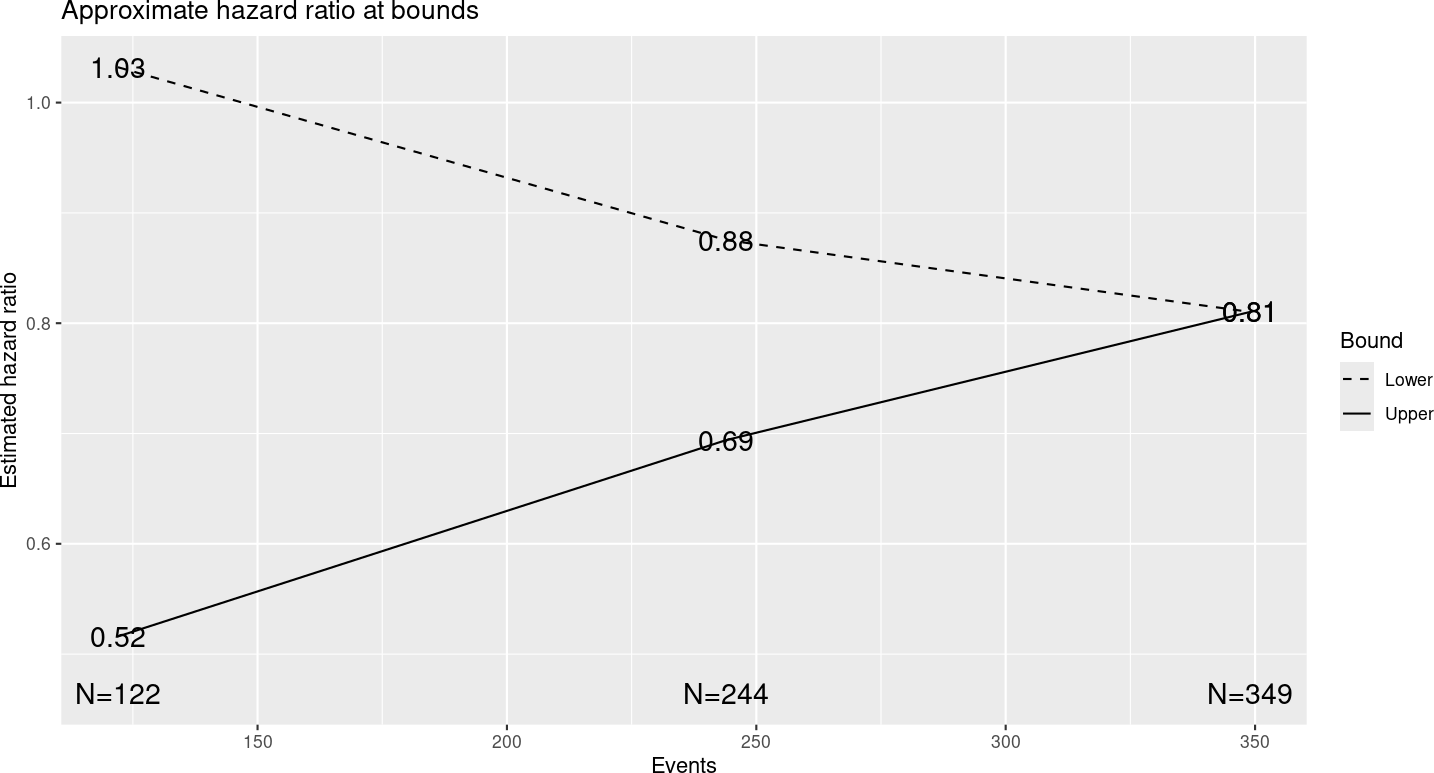### 4.2.3 Text output

The following is a direct copy of output for the group sequential design from the ‘Text’ output tab. As you can see, this is fairly lengthy and perhaps less readable than the plot or tabular output. However, it does provide complete information on enrollment rates in addition to what we have seen in other output.

Time to event group sequential design with HR= 0.6
Equal randomization:          ratio=1
Asymmetric two-sided group sequential design with
90 % power and 2.5 % Type I Error.
Upper bound spending computations assume
trial continues if lower bound is crossed.

----Lower bounds----  ----Upper bounds-----
Analysis  N    Z   Nominal p Spend+  Z   Nominal p Spend++
1  57 -0.69    0.2437 0.0044 3.71    0.0001  0.0001
2 114  1.00    0.8419 0.0396 2.51    0.0060  0.0059
3 170  1.99    0.9769 0.0560 1.99    0.0231  0.0190
Total                     0.1000                 0.0250
+ lower bound beta spending (under H1):
Lan-DeMets O'Brien-Fleming approximation spending function with none = 1.
++ alpha spending:
Lan-DeMets O'Brien-Fleming approximation spending function with none = 1.

Boundary crossing probabilities and expected sample size
assume any cross stops the trial

Upper boundary (power or Type I Error)
Analysis
Theta      1      2      3  Total  E{N}
0.0000 0.0001 0.0059 0.0173 0.0233 107.7
0.2562 0.0372 0.5473 0.3155 0.9000 131.7

Lower boundary (futility or Type II Error)
Analysis
Theta      1      2      3  Total
0.0000 0.2437 0.5998 0.1333 0.9767
0.2562 0.0044 0.0396 0.0560 0.1000
T        n    Events HR futility HR efficacy
IA 1  16.64612 136.4612  56.50599       1.203       0.373
IA 2  24.39108 213.9108 113.01190       0.828       0.623
Final 32.95685 239.5685 169.51788       0.736       0.736
Accrual rates:
Stratum 1
0-2           2.5
2-4           5.0
4-6           7.5
6-26.96      10.0
Control event rates (H1):
Stratum 1
0-Inf      0.12
Censoring rates:
Stratum 1
0-Inf      0.01

The ‘Text’ tab also is the only place where you can find the sample size required for fixed design without interim analyses: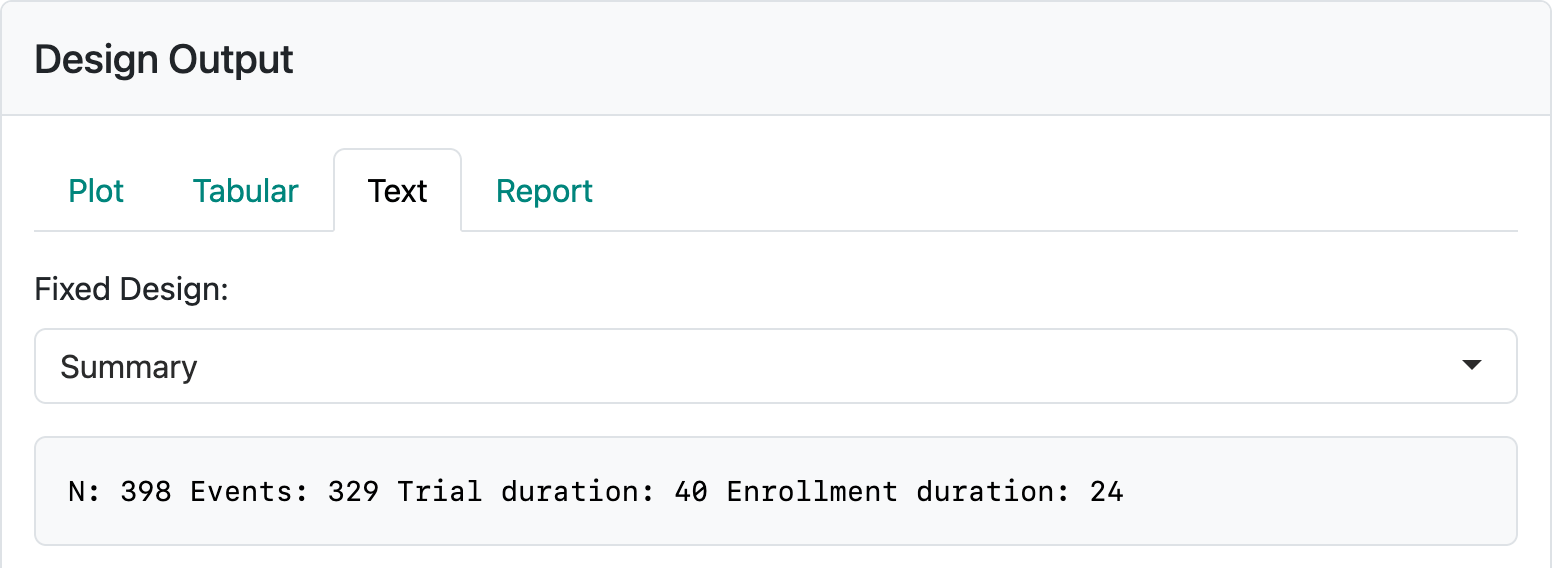In this case, you can see a sample size of 229 enrolled over 25.9 months with a 6-month follow-up period (total study time of 25.9 + 6 = 31.9 months) and 161 endpoint events for analysis is required. In actually executing the trial, the time to get to the desired enrollment or event count may vary; the final event count of 161 should drive the timing of the analysis.

## 4.3 Making a report

We consider a modification of the default design, generate an R markdown file based on that design, then modify the design to ensure an integer event counts at analyses are planned as well as an integer sample size that is even for 1:1 randomization or a multiple of ‘r + 1’ for ‘r:1’ randomization.

Open the app to the default design ‘Endpoint type’ set to ‘Time-to-event’. Set the hazard ratio to power the trial at 85% with an assumed hazard ratio of 0.7. Change the enrollment duration to 24 months and total study duration to 36 months. Change the dropout rate to 0.005 which translates to about a 6% dropout rate per year. Set the information fraction at interim analyses to 0.65 and 0.85. Set timing of the interim analyses at 40% and 85% information fraction.

For interim spending we use the default choice of ‘No parameters’ and O’Brien-Fleming-like for efficacy and a custom 2-parameter spending function for futility (Keaven M Anderson and Jason B Clark16). Specifically, we choose a Cauchy spending function with the proportion of $$\beta$$-spending at 50% and 54.6% at the first and second interim analyses. The spending functions are plotted below.## 4.4 Exercises

1. Download and open the file tte-integer-design.Rmd. Run the report and review the output.
2. Play with the ‘Update’ tab to set interim and final analysis options in line with previous sections. It will be useful to ensure you know the behavior of designs evaluated with integer event counts as well as how to ensure use of all $$\alpha$$ at the final analysis and not over-spending at interim analyses to ensure there is $$\alpha$$ left for the final analysis if this is what is desired.